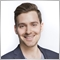# Find bar of the same time one day ago575

Hi guys,

As described in the title, I want to find the bar of one day ago at exactly the same time. So e.g. the time now is 20:23 on the 06.10.2017 which is covered by the 20:20 M5-bar. I want to find the M5 that covers yesterday's (05.10.2017) period of 20:20. How would you do it?

Thanks :)20214

1. You probably do not want yesterday (weekends and market holidays.) You want trading days.
2. Save the time, find yesterday date, find the correct time.
```#define HR2400 (PERIOD_D1 * 60)  // 86400 = 24 * 3600 = 1440 * 60
#define INDEX   uint
#define SECONDS uint
SECONDS     time(datetime when=0){        if(when == 0) when = TimeCurrent();
return SECONDS(when % HR2400);
}
datetime    date(datetime when=0){        if(when == 0) when = TimeCurrent();
return datetime(when - time(when) );
}
datetime    tomorrow( datetime when=0){   return date(when) + HR2400;          }
datetime    yesterday(datetime when=0){
datetime  BOD  = date(when);
INDEX    iBod  = iBarShift(_Symbol, _Period, BOD);
return date(Time[iBod-1]);
}
:
datetime when         = TimeCurrent();
SECONDS  TodayTOD     = time(when);
datetime yesterdayBod = yesterday(when);
INDEX    yesterdayTOD = iBarShift(_Symbol, _Period, yesterday + TOD);```
3. The other approach is to use the D1 chart, but you must must handle 4066/4073 errors.575

Thank you whroeder1, I think it will work just fine (I'll test it tomorrow). There's no way working around that complexity, is there ;)575

Samuel Beer:

Thank you whroeder1, I think it will work just fine (I'll test it tomorrow). There's no way working around that complexity, is there ;)

So I tested it doesn't quite work, but I can't understand why. Something is fishy with unix time...

I have a Log function which logs Date of Day and Time of Day when OnCalculate is called.

```int OnCalculate(const int rates_total,
const int prev_calculated,
const datetime &time[],
const double &open[],
const double &high[],
const double &low[],
const double &close[],
const long &tick_volume[],
const long &volume[],
{
Print("Date of Day time[rates_total-1-0) = ", TimeToStr(DateOfDay(time[rates_total-1-0])), " time into day = ", TimeToStr(TimeOfDay(time[rates_total-1-0]), TIME_SECONDS));

...
}```

Now I would've expected it to log the date of the day of the first bar from the back of the chart and the time in seconds that we've already progressed into that day at this point. So what I expected was:

Date of Day time[rates_total-1-0) = 2014.06.12 00:00 time into day = 14:30:00

What actually prints though is:

Date of Day time[rates_total-1-0) = 2014.06.12 08:30 time into day = 06:00:00

The day doesn't start at midnight, and TimeOfDay() is also off therefore. It seems like the linearity of unix time is broken somewhere and the seconds do not add up to all full days since 1970.1.1 ... How can this even be?20214